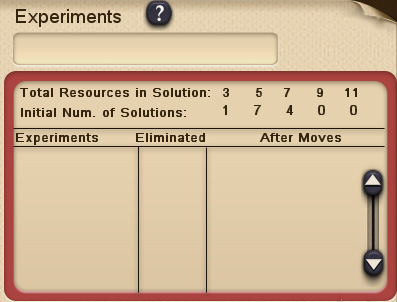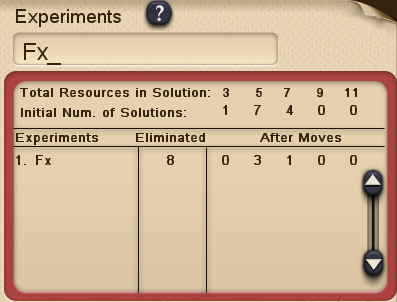### Entering Experiments

On the right half of the MSQ screen is the section for Experiments. The user is given initial data on the different Solutions in each Quest including the total number of different Solutions as well a breakdown of the number of Solutions by length. Length means how many Resources symbols are used in the Solution, either 3,5,7,9,11 or 13 symbols. Since the operations used in basic MSQ are all binary (that is, they operate on two numbers), Solutions in basic MSQ will have an odd number of Resources symbols. The number of numerals will always be one more than the number of operation signs. Parentheses are not counted.The Experiment data chart for our sample Quest looks like this:

Goal: 6     Resources: - - x / / 1 2 3 4
This Quest has 12 different Solutions.
Experiments ?

Total Resources in Solution: 3 5 7 9 11
Initial Num. of Solutions:      1 7 4 0 0

This data chart shows that there is 1 Solution that uses three symbols from the Resources, there are 7 Solutions that use five Resources symbols and 4 Solutions that use seven Resources symbols. An Experiment consists of creating a constraint expression that will Forbid or Require certain Resources symbols to see what the effect will be on the remaining number of Solutions of each length. To enter an Experiment, the user should click in the Experiment text box and then use the keypad at the bottom to type F (followed by any symbols the user wishes to forbid the use of) and/or R (followed by any symbols the user wishes to require the use of in every Solution), and then click on the ENTER button.

For example: The experiment created by typing: Fx has the following result.

Goal: 6     Resources: - - x / / 1 2 3 4
This Quest has 12 different Solutions.This experiment shows that when we are forbidden to use the x sign in Resources for Solutions, the effect is that 8 of the total twelve Solutions are eliminated and that no Solutions remain using three symbols, 3 Solutions using five symbols remain, and 1 Solution remains using seven symbols. Careful analysis of the results of experiments can tell you much about the actual symbols used in the undiscovered Solutions.

The experiment FxR// yields the result:
Goal: 6     Resources: - - x / / 1 2 3 4This Quest has 12 different Solutions.
This experiment shows that forbidding the multiplication sign and requiring two division signs will eliminate 9 Solutions leaving 2 Solutions of length five and 1 Solution of length seven. It is useful to print out or create a worksheet that lists spaces for the operations and numerals used in various length Solutions. That way when one discovers that, for example two Solutions of length five use two divisions signs, then one can write them in the spaces for operations in two of the undiscovered Solutions of length five. Filling in the spaces with the actual operations and numerals used makes it easier to deduce patterns and figure out the undiscovered Solutions.